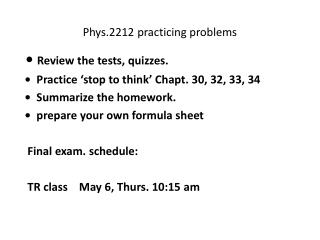DownloadDownload PresentationPhys.2212 practicing problems

# Phys.2212 practicing problems

Télécharger la présentation## Phys.2212 practicing problems

- - - - - - - - - - - - - - - - - - - - - - - - - - - E N D - - - - - - - - - - - - - - - - - - - - - - - - - - -
##### Presentation Transcript

1. Phys.2212 practicing problems • Review the tests, quizzes. • Practice ‘stop to think’ Chapt. 30, 32, 33, 34 • Summarize the homework. • prepare your own formula sheet Final exam. schedule: TR class May 6, Thurs. 10:15 am

2. 1. Homework 28.39. A hollow metal sphere has inner radius a and outer radius b . The hollow sphere has charge +3Q . A point charge +Q sits at the center of the hollow sphere. • Determine the electric fields E in the three region r ≤ a, a<r<b • And r ≥ b. • (ii) How much charges is on the inside surface of the hollow sphere? On the exterior surface. • (iii) Determine the electric potential V in the three region r ≤ a, a<r<b and r ≥ b. (define V = 0 at r → ∞)

3. In above problem, if a = 3cm, b = 4cm, Q = 1 nC, make plots ofE vs r and V vs r

4. 2. Example 29.7 (P895) A proton in a capacitorA parallel-plate capacitor is constructed of two 2.0-cm-diameter disks spaced 2.0 mm apart. It is charged to a potential difference of 500 V .

5. In above problem • (i) What is the electric field strength inside? • (ii) How much charge is on each plate? • (iii) What is the capacitance ? • (iii) A proton is shot through a small hole in the negative plate with a speed of 2.0x105 m/s. Does it reach the other side? If not where is the turning point?

6. In above problem, make plots of E vs x and V vs x( we set origin x = 0 at V=0 point)

7. 3. Homework 30.64 (P938)What is the capacitance of the three concentric metal spherical shells in the Figure?

8. 4. Homework 32.46 (p995)What are the emf and internal resistance of the battery in the figure

9. 5. Homework 32.73 (p997) The capacitor in Figure begins to charge after the switch closes at t = 0sa. What is ΔVc a very long time after the switch has closed?b. What is Qmax in terms of ε, R, C?c. In this circuit, does I = + dQ/dt or –dQ/dt?D. Find as expression for the current I at time t. Graph I from t =0 to t= 5s.

10. 6. Example 33.11 ( P1020) The radius of cyclotron motionan electron is accelerated from rest through a potential difference of 500 V, then ejected into a uniform magnetic field. Once in the magnetic field, it completes half revolution in 2.0ns. What is the radius of its orbit ( the electron charge-mass ratio q/m = 1.76x1011)Does the magnetic force do any work?

11. 7. Two very long fixed wires cross each other perpendicularly. They do not touch but are close to each other as shown. Equal currents flow in the wires, in the directions shown. Find the magnetic field B at position A, which has the equal perpendicular distance to each wire. Then you try to indicate on diagram the location of points where the net magnetic field is zero. Explain your answer.

12. 8. Homework 34.38:The rectangular loop in Figure has 0.02 Ω resistance, (i) the induced current in the loop at this instant is cw or ccw(ii) derive the induced emf is: • Here a, b, c refer to text book (p1051) Fig 34.18 • (iii) What is the induced current in the loop at this instant? b V c a

13. 9.A diverging lens of focal length 3.0 cm is placed 8.0 cm from an object which is 45 mm tall • (i) draw the ray diagram and determine where is the image? • (ii) use equations to calculate where is the image and how tall it will be • (iii) Is the image real or virtual? Is the image upright or inverted?

14. 10. As you did in the lab , this is a series RLC AC circuit.We have C = 0.005 μF, L = 25 mH, R = 100Ω(a) What is the resonance frequency fo (1.42x104 Hz)(b) suppose the AC ε (rms) = 110V, what is the current I (rms) at resonance frequency(c) If you had used a larger or small resistor would be resonant frequency have changed? Why or why not, Would anything else about your results have changed?